# using the following information to calculate the expected return and the standard deviation of a portfolio...

 using the following information to calculate the expected return and the standard deviation of a portfolio that is 50% in vested in 3 door inc. and in down co. 3 doors inc. down co. expected return 14% 10% standard deviation 42 31 correlation 0.1 What is the standard devciation if the correlation is +1, 0, and -1 here. What do you see happening to the portfolio volatility and why?

This Homework Help Question: "using the following information to calculate the expected return and the standard deviation of a portfolio..." No answers yet.

We need 3 more requests to produce the answer to this homework help question. Share with your friends to get the answer faster!

0 /3 have requested the answer to this homework help question.

Once 3 people have made a request, the answer to this question will be available in 1-2 days.
All students who have requested the answer will be notified once they are available.
##### Add Answer of: using the following information to calculate the expected return and the standard deviation of a portfolio...
Similar Homework Help Questions
• ### Use the following information to calculate the expected return and standard deviation of a portfolio that...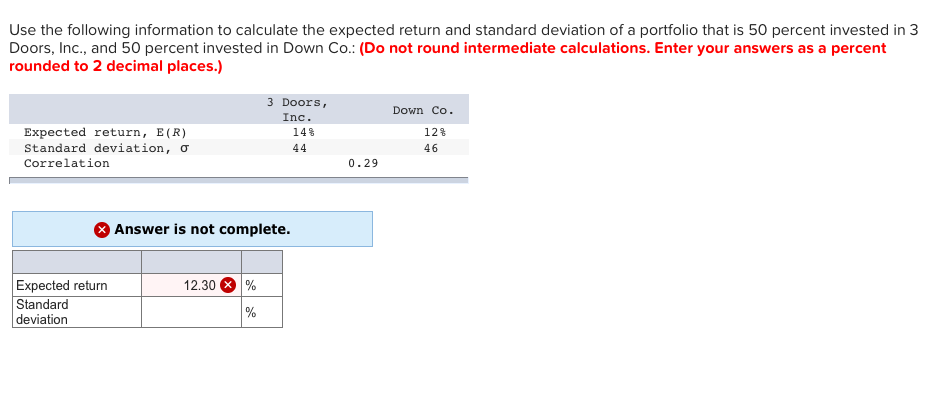Use the following information to calculate the expected return and standard deviation of a portfolio that is 50 percent invested in 3 Doors, Inc., and 50 percent invested in Down Co.: (Do not round intermediate calculations. Enter your answers as a percent rounded to 2 decimal places.) 3 Doors, Inc. 143 44 Expected return, E(R) Standard deviation, o Correlation Down Co. 12% 46 0.29 Answer is not complete. 12.30 % Expected return Standard deviation

• ### Use the following information to calculate the expected return and standard deviation of a portfolio that...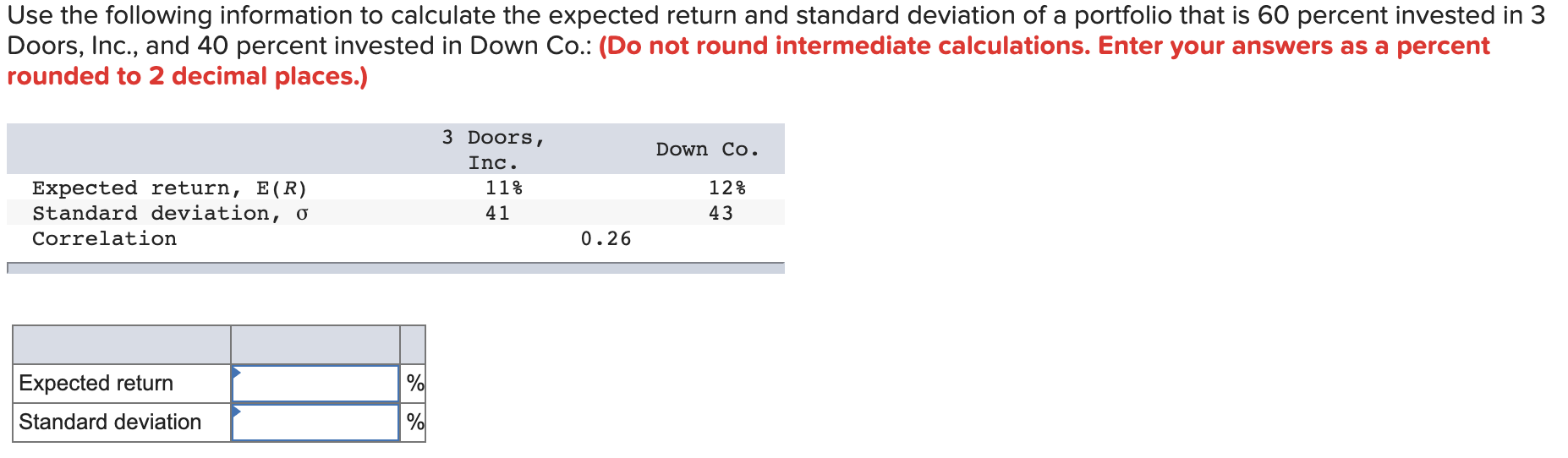Use the following information to calculate the expected return and standard deviation of a portfolio that is 60 percent invested in 3 Doors, Inc., and 40 percent invested in Down Co.: (Do not round intermediate calculations. Enter your answers as a percent rounded to 2 decimal places.) 3 Doors, Inc. 11% 41 Expected return, E(R) Standard deviation, 0 Correlation Down Co. 12% 43 0.26 Expected return Standard deviation doo

• ### Problem 11-12 Use the following information to calculate the expected return and standard deviation of a...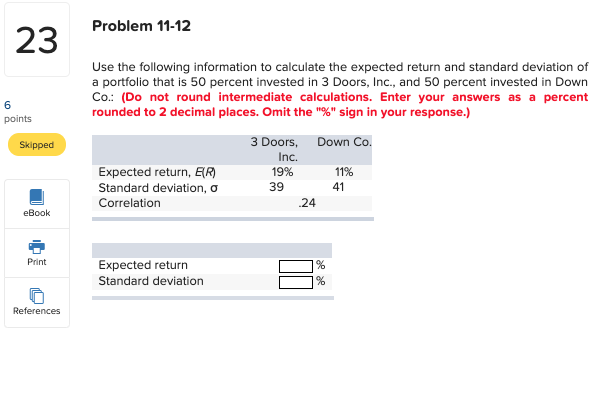Problem 11-12 Use the following information to calculate the expected return and standard deviation of a portfolio that is 50 percent invested in 3 Doors, Inc., and 50 percent invested in Down Co.: (Do not round intermediate calculations. Enter your answers as a percent rounded to 2 decimal places. Omit the "%" sign in your response.) points Skipped 3 Doors Down Co. Inc. 19% 11% 39 41 Expected return, ER) Standard deviation, o Correlation 24 * eBook Print Expected return...

• ### Refer to the table below: Down Co. 3 Doors, Inc. 14% 42 10% Expected return, E(R)...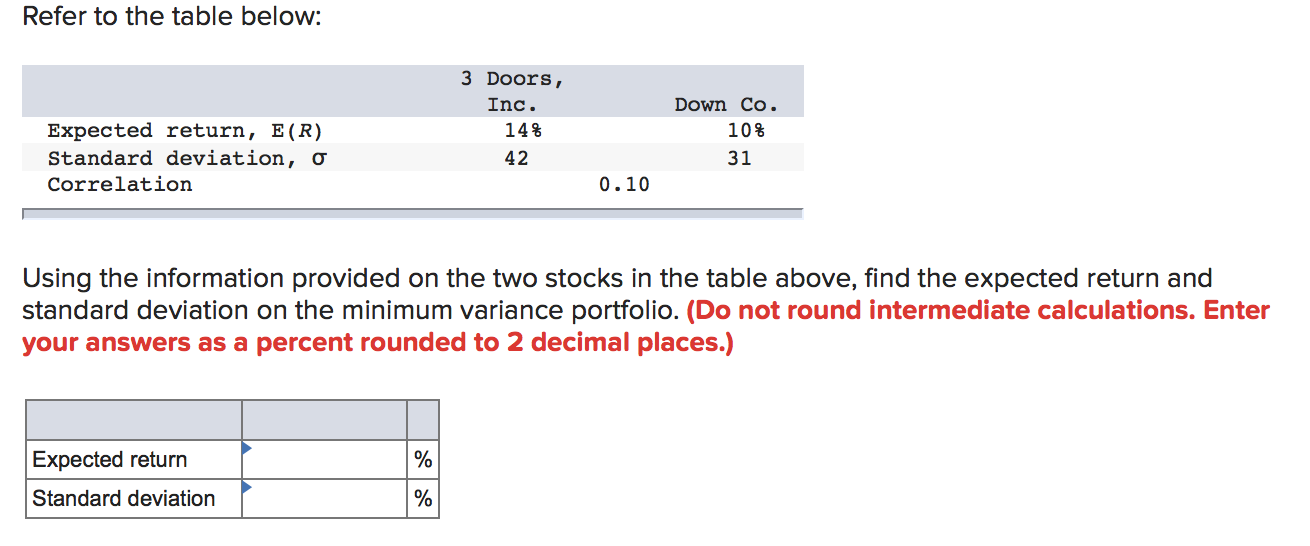Refer to the table below: Down Co. 3 Doors, Inc. 14% 42 10% Expected return, E(R) Standard deviation, o Correlation 31 0.10 Using the information provided on the two stocks in the table above, find the expected return and standard deviation on the minimum variance portfolio. (Do not round intermediate calculations. Enter your answers as a percent rounded to 2 decimal places.) Expected return Standard deviation

• ### Refer to the table below: 3 Doors, Inc. 215 Down Co Expected return, E(R) Standard deviation,...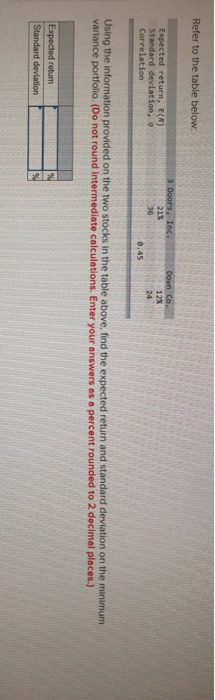Refer to the table below: 3 Doors, Inc. 215 Down Co Expected return, E(R) Standard deviation, o Correlation Using the information provided on the two stocks in the table above, find the expected return and standard deviation on the minimum variance portfolio. (Do not round Intermediate calculations. Enter your answers as a percent rounded to 2 decimal places.) Expected return Standard deviation

• ### Need another explanation of correlation

The expected return and standard deviation of a portfolio that is 50 percent invested in 3 Doors, Inc., and 50 percent invested in Down Co.3 Doors, Inc.Down Co.Expected return, E (R)19%11%Standard deviation, s5236What is the standard deviation if the correlation is +1? 0? -1? (Round your answer to 2 decimal places. Omit the "%" sign in your response.)Correlation +1:%Correlation 0:%Correlation -1:%

• ### 8. Calculate the PORTFOLIO Expected Return and standard deviation of a 60/40 Portfolio of Asset A...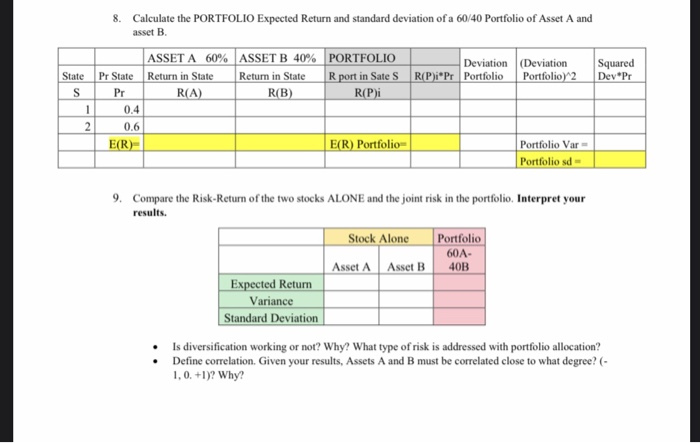8. Calculate the PORTFOLIO Expected Return and standard deviation of a 60/40 Portfolio of Asset A and asset B. ASSET A 60% ASSET B 40% Return in State Return in State R (A) R(B) PORTFOLIO Rport in Sate S R(P)i Deviation R(P)i Pr Portfolio (Deviation Portfolio 2 State S Squared Dev*Pr Pr State P 0.4 0.6 E(R) E(R) Portfolio Portfolio Var Portfolio sd - 9. Compare the Risk-Return of the two stocks ALONE and the joint risk in the portfolio...

• ### Assume that the assumptions of the CAPM hold. The expected return and the standard deviation of...

Assume that the assumptions of the CAPM hold. The expected return and the standard deviation of the market portfolio are 7% and 14%, respectively. There are two individual stocks A and B: Mean Return A: 4% Standard Deviation A: 18% Mean Return B: 12% Standard Deviation B: 36% Stock A has a correlation of 0.2 with the market portfolio. A.What is the beta of stock A? B.What is the risk free rate? C.What is the beta of a portfolio with...

• ### Correlation

The expected return and standard deviation of a portfolio that is 60 percent invested in 3 Doors, Inc., and 40 percent invested in Down Co.3 Doors, Inc.Down Co.Expected return, E (R)16%11%Standard deviation, s4934.5What is the standard deviation if the correlation is +1? 0? -1? (Round your answer to 2 decimal places. Omit the "%" sign in your response.)Correlation +1:%Correlation 0:%Correlation -1:%

• ### Given the following information, calculate the expected return and standard deviation for a portfolio that has...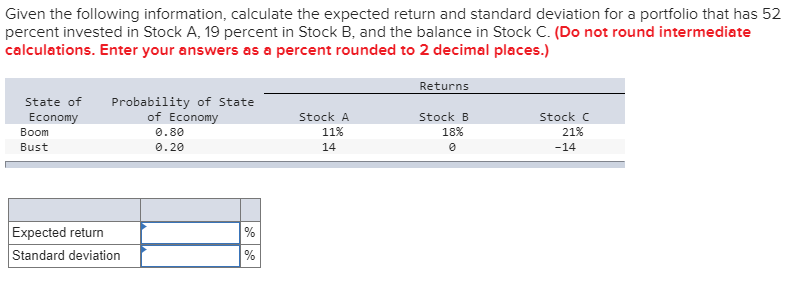Given the following information, calculate the expected return and standard deviation for a portfolio that has 52 percent invested in Stock A, 19 percent in Stock B, and the balance in Stock C. (Do not round intermediate calculations. Enter your answers as a percent rounded to 2 decimal places.) Returns State of Economy Boom Bust Stock C Probability of State of Economy 0.80 0.20 Stock A 11% 14 Stock B 18% 21% -14 Expected return Standard deviation

Need Online Homework Help?